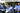# CBSE Class 10 science paper 2018 analysisCBSE class 10 science paper 2018 was conducted today on March 16, 2018.

The question paper was divided into two parts A and B and there were total 27 questions.

Part A had 21 questions and skill-based Part B, had 6 questions.

Section A

• Question no. 1 and 2 were of 1 marks each.
• Question number 3 – 5 were of 2 marks each.
• Question number 6 to 15 were of 3 marks each.
• Question no 16 – 21 were of 5 marks each.

Total Marks: 68

Section B

• In this section, there were total six questions each carrying 2 marks.
• All these questions were based on practical skills.

Total Marks : 12

– Cbse board question papers for class 12 –

## Marking Scheme for CBSE Class 10 Science Paper 2018

 Chapter Name Weightage in terms of marks (2017) Weightage in terms of marks (2018) Light Reflection and Refraction 21 10 Human Eye and Colourful World 6 5 Electricity 0 11 Magnetic Effects of Electric Current 0 5 Sources of Energy 1 1 Chemical Reactions and Equations – 3+2* = 5 Acids, Bases and Salts 1* 3 Metals and Non-metals 1* 5+2* = 7 Carbon and its compounds 1+3+3+5+1*+2* = 15 2+3 = 5 Periodic Classification of Elements 3+3=6 5 Life Processes 0 7 Control and Coordination 0 3 How do Organisms Reproduce? 17 10 Heredity and Evolution 13 1 Our Environment 1 3 Management of Natural Resources 7 3